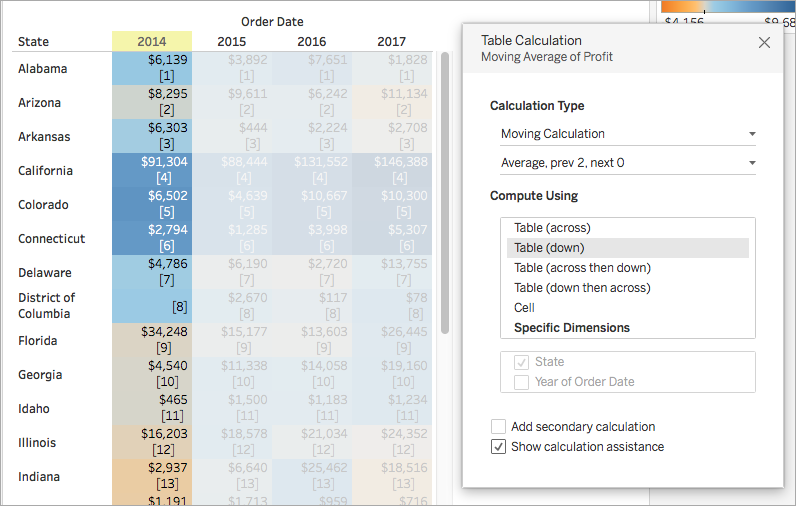Quick table calculations allow you to quickly apply a common table calculation to your visualisation using the most typical settings for that calculation type. This article demonstrates how to apply a quick table calculation to a visualisation using an example.

The following quick table calculations are available in Tableau for you to use:

• Running total
• Difference
• Percent difference
• Percent of total
• Rank
• Percentile
• Moving average
• YTD total
• Compound growth rate
• Year of year growth
• YTD growth

## How does a quick table calculation differ from a table calculation?

Quick table calculations are table calculations that you can apply quickly to your visualisation in Tableau. They are applied to the visualisation with the most typical settings for the calculation type you choose so that you can continue on with your analysis. With traditional table calculations, you can apply the same settings, but you must apply them manually.

## Apply a quick table calculation to the visualisation

Follow along with the steps below to learn how to apply a quick table calculation to a visualisation.

### Set up the visualisation

1. Open Tableau Desktop and connect to the Sample-Superstore data source, which comes with Tableau.

2. Navigate to a new worksheet.

3. From the Data pane, under Dimensions, drag Order Date to the Columns shelf.

4. From the Data pane, under Dimensions, drag State to the Rows shelf.

5. From the Data pane, under Measures, drag Sales to Text on the Marks Card.

6. From the Data pane, under Measures, drag Profit to Colour on the Marks Card.

7. On the Marks card, click the Mark Type drop-down and select Square.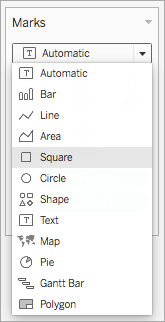The visualisation updates to look like this: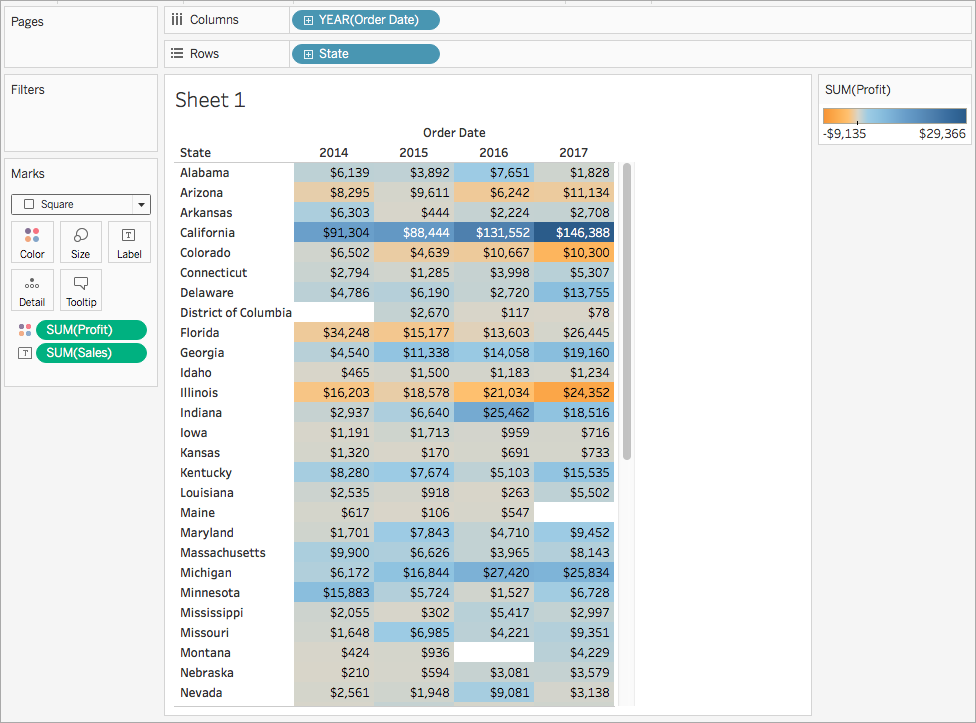### Apply the quick table calculation

1. On the Marks card, right-click SUM(Profit) and select Quick Table Calculation > Moving Average.

Note: You can only perform quick table calculations on measures in the view.

A delta symbol appears on the field to indicate that a quick table calculation is being applied to the field. The colours in the visualisation update to show the moving average of profit across the years.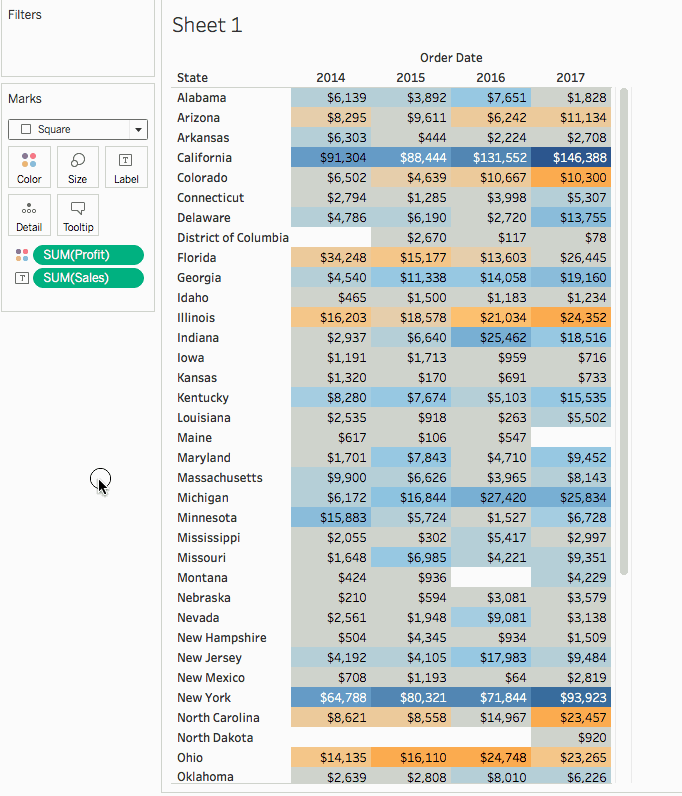### (Optional) Customise the quick table calculation

1. On the Marks card, right-click Sum(Profit) and select Edit Table Calculation.

2. In the dialog box that opens, you can configure the following options:

• The calculation type

• How to aggregate the values

• How to compute the calculation (how to address and partition the calculation)

The visualisation updates as you make changes to the calculation. Highlighting and numbering are used to demonstrate how the calculation is being computed. For example, in the following image, the calculation is being computed across the table, for each State.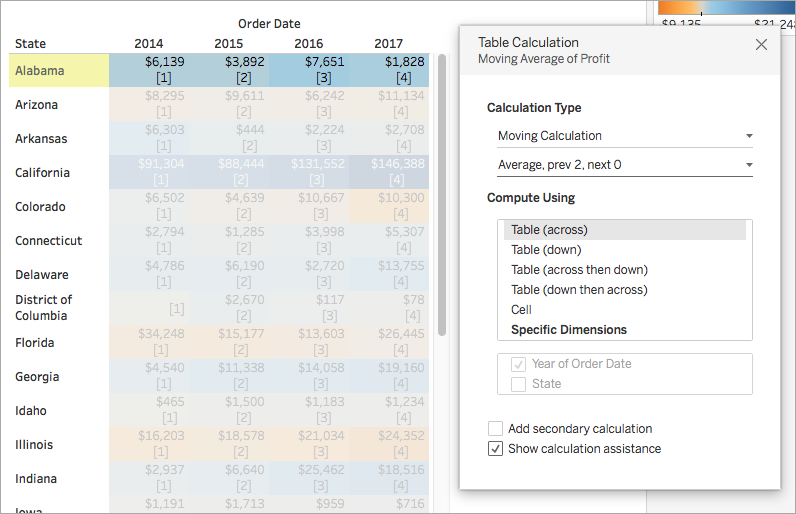If this setting is changed, the visualisation and highlighting update to indicate the change.